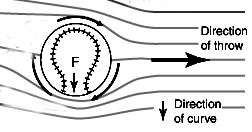Curve Ball

Throw Him a Curveball

Introduction

Figuring out the direction that a spinning baseball will curve is an excellent test of your understanding of the Bernoulli equation.Is this diagram correct?

Bernoulli's equation does not use the relative velocity between the air flowing over the ball and the surface of the ball!

It uses the change in speed of the ball between two points along a streamline.

Consider the streamline that goes over the top of the ball. Far to the right the streamline is at atmospheric pressure, As it flows over the top of the ball it interacts with the seams of the ball and slows down the pressure increases above atmospheric pressure.

Below the ball the streamline at the far right is also at atmospheric pressure. It interacts with the seams and speed sup beneath the baseball, the pressure decreases. The difference in pressure between top and bottom makes the ball curve down in the image which is correct.

If the relative velocity between the ball and the air mattered then the ball would curve the other way since the relative velocity above the ball is faster and that below the ball is slower.

 Scientific Explorations with Paul Doherty © 2004 6 February 2004